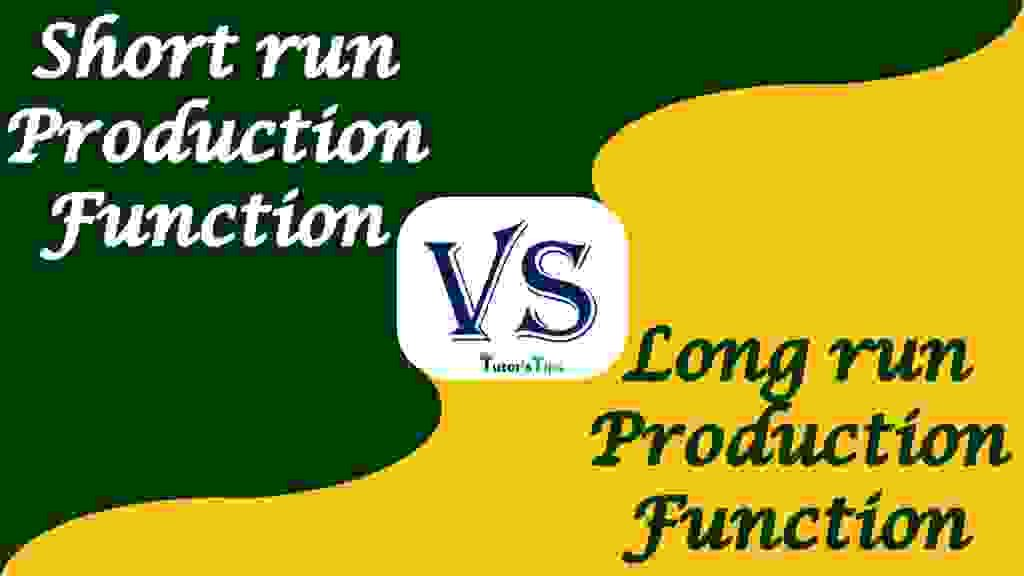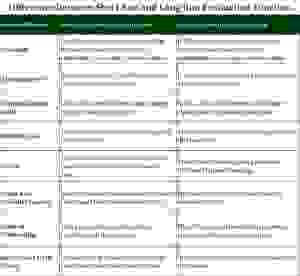# Difference between Short Run and Long Run Production FunctionThe main difference between short-run and long-run production function is that in on run, the producer is not able to increase or decrease the quantity of all inputs Whereas in long run, the quantity of all inputs can be changed.

To know the difference between these two, we must clear the meaning of these terms:

## Meaning of Short-Run Production Function:

Short-run is a period in which the output can be increased by increasing the input of some variable factor. In the short run, the fixed factor remains constant and variable factors change with change in output.

For this, When one factor is a fixed factor and other is variable, then the function can be expressed as:

Qx = f(L, K¯)

Here,

Q= Output of commodity-X

L = Labour, a variable factor

K = Capital, a fixed factor

In this, the output can only be increased by increasing the application of variable factors i.e. labour.

### Illustration:

We can understand this with an illustration:

Suppose, A  producer uses 10 units of capital as a fixed factor and 10 units of labour as a variable factor to produce 50 units of commodity as output.

50x = f(10L, 10K¯)

And, If the output is increased from 50 units to 60 units by adding more labour to 15 units. It will be the production function for the short run.

60x = f(15L, 10K¯)

Since Capital is constant and only labour changes, the ratio between capital and labour tends to change. This generates the law of variable proportion.

## Meaning of Long run Production Function:-

Long Run is a period in which the output can be increased by increasing all the inputs. In the long run, all the factors are variable and change with change in output.

For this, When both factors are variable, then the production function can be expressed as:

Qx = f(L, K)

Here,

Q= Output of commodity-X

L = Labour, a variable factor

K = Capital, a variable factor

In long-run function, the output can be increased by increasing the application of variable factors i.e. labour and capital.

### Illustration:

We can understand this with an illustration:

Suppose, A  producer uses 5 units of capital as a fixed factor and 10 units of labour as a variable factor to produce 50 units of commodity as output.

50x = f(10L, 5K)

And, If the output is increased from 50 units to 100 units by adding more labour and capital to 20 and 10 units respectively. Then, It will be the production function for the short run. It can be written as:

100x = f(20L, 10K)

Since Capital and labour, both changes to increase productivity, the ratio between capital and labour doesn’t change. This generates the law of constant proportion.

## Chart of Difference :

Basis of Difference

Short-Run Production Function

Long-Run Production Function

Meaning It defines the functional relationship between inputs and output of a commodity for a short period of time.  It defines the functional relationship between inputs and output of a commodity for a longer period of time.

### Also known as

It is also known as Variable proportion type of production function. It is also known as a fixed proportion type of production function.

### Capital-Labour Ratio

In this, the capital-labour ratio changes with the change in output. In this, the capital-labour ratio doesn’t change with the change in output.

Related Law

The law of returns to a factor is applied for this function. The law of returns to a scale is applied for this function.

Curve

The curve of the short-run production function is parallel to the horizontal axis. The curve of the long-run production function is upward sloping.

Fixed and Variable Factors

Here, the capital is assumed as the fixed factor and labour as a variable factor.

Here, all the factors of production are variable factors.

Scale of Production

There is no change in the scale of production in the short run.

Here, The scale of production is always changed with a change in output.

Entry and Exit of Firms

In this, there are barriers for firms to enter and to shut down but cant exit.

In this, the firms are free to enter and exit the market.

Expression

Qx = f(L, K¯)

Qx = f(L, K)

## Download the chart:-

If you want to download the chart, please download the following image and PDF file:-Chart of differenceChart of Difference

## Conclusion:

Thus, To understand the whole concept of the production function, it is classified into the short-run and long-run. In the shorter period of time, the capital inputs are assumed as fixed and others are variable whereas all the inputs are assumed as the variable for a longer period of time.

Thanks Please share with your friends

Comment if you have any question.

Check out Business Economics Books @ Amazon.in

This site uses Akismet to reduce spam. Learn how your comment data is processed.